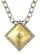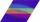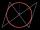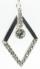# Box

Cardboard box shaped quadrangular prism with a rhombic base. Rhombus has a side 5 cm and one diagonal 8 cm long and height of the box is 12 cm. The box will open at the top.

How many cm2 of cardboard we need to cover overlap and joints that are 5% of area of cardboard?

Result

S =  277.2 cm2

#### Solution:Leave us a comment of this math problem and its solution (i.e. if it is still somewhat unclear...):Be the first to comment!## Next similar math problems:

1. Cardboard boxWe want to make a cardboard box shaped quadrangular prism with rhombic base. Rhombus has a side of 5 cm and 8 cm one diagonal long. The height of the box to be 12 cm. The box will be open at the top. How many square centimeters cardboard we need, if we cal
2. JewelRhombus-shaped jewel have area of 93 mm2 and the edge in long 13.2 mm. Calculate the size of rhombus acute angle.
3. PoolMr. Peter build a pool shape of a four-sided prism with rhombus base in the garden. Base edge length is 8 m, distance of the opposite walls of the pool is 7 m. Estimated depth is 144 cm. How many hectoliters of water consume Mr. Peter to fill the pool?
4. PrismThe base of the prism is a rhombus with a side 30 cm and height 27 cm. The height of the prism is 180% longer than the side length of the rhombus. Calculate the volume of the prism.
5. PrismCalculate the volume of the rhombic prism. Base of prism is rhombus whose one diagonal is 47 cm and the edge of the base is 28 cm. The edge length of the base of the prism and height is 3:5.
6. AnglesDetermine the interior angles of a rhombus with area 319.1 cm2 and perimeter 72 cm.
7. Rhombus HPCalculate area of the rhombus with height 24 dm and perimeter 12 dm.
8. RhombusCalculate the perimeter and area of ​​a rhombus whose diagonals are 38 cm and 55 cm long.
9. Maximum area of rhombusCalculate the interior angles at which equilateral rhombus has a maximum area.
10. RhombusFind the length of the other diagonal and area of rhombus. The perimeter of a rhombus is 40 cm and one of the diagonals is of length 10 cm.
11. Rhombus 2Calculate the area of rhombus which has a height v=48 mm and shorter diagonal u = 60 mm long.
12. ParallelogramCalculate area of the parallelogram ABCD as shown if |AB| = 19 cm, |BC| = 18 cm and angle BAD = 90°
13. Circle in rhombusIn the rhombus is inscribed circle. Contact points of touch divide the sides to parts of length 19 cm and 6 cm. Calculate the circle area.
14. Trapezoid MO-5-Z8ABCD is a trapezoid that lime segment CE divided into a triangle and parallelogram as shown. Point F is the midpoint of CE, DF line passes through the center of the segment BE and the area of the triangle CDE is 3 cm2. Determine the area of the trapezoid A
15. Rhombus diagonalArea of rhombus is 224. One diagonal measures 33, find length of other diagonal.
16. Diagonals in the diamondThe length of one diagonal in diamond is 24 cm greater than the length of the second diagonal and diamond area is 50 m2. Determine the sizes of the diagonals.
17. Rhombus problemPerimeter of the rhombus is 204 dm and it area is 2244 dm2. Calculate the height of the rhombus.